xAbsolute electrode potentialEncyclopedia
Absolute electrode potential, in electrochemistry
Electrochemistry
Electrochemistry is a branch of chemistry that studies chemical reactions which take place in a solution at the interface of an electron conductor and an ionic conductor , and which involve electron transfer between the electrode and the electrolyte or species in solution.If a chemical reaction is...

, according to an IUPAC definition, is the electrode
Electrode
An electrode is an electrical conductor used to make contact with a nonmetallic part of a circuit...

potential of a metal measured with respect to a universal reference system (without any additional metal-solution interface).

## Definition

According to a more specific definition presented by Trasatti, the absolute electrode potential is the difference in electronic energy between a point inside the metal (Fermi level
Fermi level
The Fermi level is a hypothetical level of potential energy for an electron inside a crystalline solid. Occupying such a level would give an electron a potential energy \epsilon equal to its chemical potential \mu as they both appear in the Fermi-Dirac distribution function,which...

) of an electrode and a point outside the electrolyte
Electrolyte
In chemistry, an electrolyte is any substance containing free ions that make the substance electrically conductive. The most typical electrolyte is an ionic solution, but molten electrolytes and solid electrolytes are also possible....

in which the electrode is submerged (an electron at rest in vacuum).

This potential is difficult to determine accurately. For this reason, standard hydrogen electrode
Standard hydrogen electrode
The standard hydrogen electrode , is a redox electrode which forms the basis of the thermodynamic scale of oxidation-reduction potentials...

is typically used for reference potential. The absolute potential of the SHE is 4.44 ± 0.02 V at 25 °C. Therefore, for any electrode at 25 °C: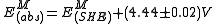where:
E is electrode potential, V
M denotes the electrode made of metal M denotes the absolute potential denotes the electrode potential relative to the standard hydrogen electrode.

A different definition for the absolute electrode potential (also known as absolute half-cell potential and single electrode potential) has also been discussed in the literature. In this approach, one first defines an isothermal absolute single-electrode process (or absolute half-cell process.) For example, in the case of a generic metal being oxidized to form a solution-phase ion, the process would be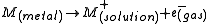For the hydrogen electrode, the absolute half-cell process would be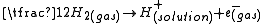Other types of absolute electrode reactions would be defined analogously.

In this approach, all three species taking part in the reaction, including the electron, must be placed in thermodynamically well-defined states. All species, including the electron, are at the same temperature, and appropriate standard states for all species, including the electron, must be fully defined. The absolute electrode potential is then defined as the Gibbs free energy for the absolute electrode process. To express this in volts one divides the Gibb’s free energy by the negative of Faraday’s constant.

Rockwood's approach to absolute-electrode thermodynamics is easily expendable to other thermodynamic functions. For example, the absolute half-cell entropy has been defined as the entropy of the absolute half-cell process defined above. An alternative definition of the absolute half-cell entropy has recently been published by Fang et al. who define it as the entropy of the following reaction (using the hydrogen electrode as an example):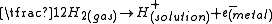This approach differs from the approach described by Rockwood in the treatment of the electron, i.e. whether it is placed in the gas phase or in the metal.

## Determination

The basis for determination of the absolute electrode potential under the Trasatti definition is given by the equation: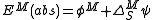where:
EM(abs) is the absolute potential of the electrode made of metal M, V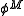is the electron work function
Work function
In solid-state physics, the work function is the minimum energy needed to remove an electron from a solid to a point immediately outside the solid surface...

of metal M, V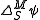is the contact (Volta) potential
Volta potential
Volta potential in electrochemistry, is the electric potential difference between two points in the vacuum:...

difference at the metal(M)-solution(S) interface, V.

For practical purposes, the value of the absolute electrode potential of the standard hydrogen electrode is best determined with the utility of data for an ideally-polarizable mercury (Hg) electrode: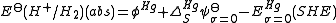where: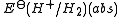is the absolute standard potential of the hydrogen electrode
σ=0 denotes the condition of the point of zero charge at the interface.

The types of physical measurements required under the Rockwood definition are similar to those required under the Trasatti definition, but they are used in a different way, e.g. in Rockwood's approach they are used to calculate the equilibrium vapor pressure of the electron gas. The numerical value for the absolute potential of the standard hydrogen electrode one would calculate under the Rockwood definition is sometimes fortuitously close to the value one would obtain under the Trasatti definition. This near-agreement in the numerical value depends on the choice of ambient temperature and standard states, and is the result of the near-cancellation of certain terms in the expressions. For example, if a standard state of one atmosphere ideal gas is chosen for the electron gas then the cancellation of terms occurs at a temperature of 296 K, and the two definitions give an equal numerical result. At 298.15 K a near-cancellation of terms would apply and the two approaches would produce nearly the same numerical values. However, there is no fundamental significance to this near agreement because it depends on arbitrary choices, such as temperature and definitions of standard states.

• Electrode potential
Electrode potential
Electrode potential, E, in electrochemistry, according to an IUPAC definition, is the electromotive force of a cell built of two electrodes:* on the left-hand side is the standard hydrogen electrode, and...

• Standard electrode potential
Standard electrode potential
In electrochemistry, the standard electrode potential, abbreviated E° or E , is the measure of individual potential of a reversible electrode at standard state, which is with solutes at an effective concentration of 1 mol dm−3, and gases at a pressure of 1 atm...

• Galvani potential
Galvani potential
Galvani potential in electrochemistry, is the electric potential difference between two points in the bulk of two phases...

• Volta potential
Volta potential
Volta potential in electrochemistry, is the electric potential difference between two points in the vacuum:...

• Electrochemical potential